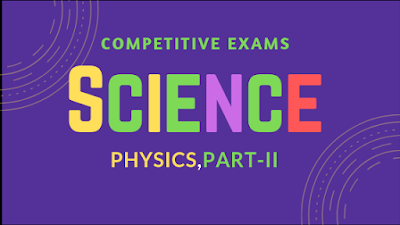# Science for Competitive Exam ,Physics part-II

Science for  Competitive Exam.GK Science

Physics for Competitive Exam part-II
Most Important MCQ type question answer for competitive exam.

Q1.Heat is a form of-
a.  Air b. Work c.  Energy d. None
Q2. 1 Calorie heat is equivalent to-
a. 4 Joule b. 4.81 Joule c. 4.18 Joule d. 4.18 J/kg
Q3.  The instrument is used to measure Temperature is-
a. Barometer b. Thermometer c. Audiometer d. None
Q4. Distance traveled by wheels of a vehicle is measured by-
a.  Odometer b. Dynamo c. Audiometer d.None
Q5. The ammeter is used to measure-
a. Voltage b. current c. Frequency d. Velocity
Q6. The intensity of Sound is Odometer by  -
a. Nanometer b. Endoscope c. Micro tom d. Audiometer
Q7. The heartbeat of the human body is measured by –
a. Cardiograph b. Cardiogram c. Endoscope d. None
Q8. ------ is a moving mechanical ladder-
a.  Tower b. Escalator c. Dynamo d. None.
Q9. Atmospheric pressure is measured by –
a.  Odometer b. Barometer c. Bolometer d. None
Q10. A device used to accelerate positively charged particles, ions etc is
a. Cyclotron b. Cryotron c. Aerometer d. Non
Q11. The speed of wind is measured by-
a.  AVO meter b. Hydrometer c.  Chronometer d. Anemometer
Q12.Purification of Milk is measured by –
a.  Lactometer b. Hydrometer c. Barometer d.None
Q13. The device used to measure the Potential difference is-
a.  Electrometer b. Electromagnet c. Electrometer.     d. None
Q14. Joule is unit of-
a. Heat b.Temperature c. Energy d. None
Q15. Heat is measured in-
a.  Ampere b. Celsius c. Joule d. Watt
Q16. Temperature is measured in –
a. Celsius b. Degree c. Joule d. None
Q17.  Mercury is used as a thermometer substance because-
a. Very costly b. Expands less than glass c. Expand 7 times more than glass,
d. None of the above
Q18. The lower fixed point of a thermometer in Fahrenheit scale is-
a.   00C    b. 32 0C   c. 320 F  d. None
Q19. The normal temperature of a human being is-
a.  370C   b. 370F   c. 320 F  d. None
Q20. The upper fixed point of a thermometer in Fahrenheit scale is
a.   2120C   b. 32 0C   c. 320 F  d.None
Q21.  The lower fixed point of a thermometer in Celsius scale is-
a.   00C   b. 32 0C   c. 320 F  d.None
Q22. The upper fixed point of a thermometer in Celsius scale is-
a.  00C   b. 100 0C   c. 320 F   d. None
Q23. The normal temperature of a teeth being is-
a 370C    b. 370F   c. 320 F   d. None
Q24. Freezing temperature of mercury is-
a.  - 390C  b. -800F  c. -420 F   d.None
Q25. A good absorber of heat is-
a.  White surface.  b. Black surface. c. Red surface.  d. None
Q26.The device used to measure the speed and direction of fighter planes is-
a. Radar,    b. Electromagnet. c. Electrometer.d. None
Q27. The device used to measure the atmospheric humidity is-
a. Hydrometer b. Electromagnet c. Electrometer. d. None
Q28 The device used to measure the extremely high temperature is-
a. Radar b. Heat scale c. Electrometer d. Pyrometer
Q29. The device used to measure the intensity of earthquake is-
a.  Seismograph b. Speedometer c. Electrometer d. None
Q30. The equipment used to measure the viscosity of the Liquid is-
a.  Thermopile b. Wavemeter.   c. Viscometer d. None
Q31. The inventor of Radar is –
a.   Bush Wall b. Robert Watson c. Galileo d.None
Q32. The inventor of Rail engine is –
a.   Marconi b. Compel c. Gorge Stefan d. None
Q33.  The inventor of E-mail is –
a.Dewar b. F.G. Otis c.  Re- Tomlinson d.None
Q34.  The inventor of Barometer is –
a.  Robert Watson b. Galileo c. Torricelli d.None
Q35.  The inventor of Steam Engine is –
a    Jeans b. J.C. Bose c. Faraday d.James Watt
Q36.  The inventor of Lift is –
a.  Newton b. Karl Benz c. F.G. Obits d.None
Q37.  The inventor of Telescope is –
a.   Bush Wall b. Hans Lippershey c. Galileo d.None
Q38.  The inventor of Transformer is –
a.  Karl Benz b. James watt c. Faraday d. None
Q39. The inventor of Radio is –
a.  Bush Wall b. Pascal c. Marconi d. J.C. Bose
Q40.  The inventor of By-cycle is –
a.  Bush Wall b. Macmillan c. Galileo d.None

ANSWER KEY: 1.a 2.c 3.b 4.a 5.b 6.d 7.a 8.b 9.b 10.a 12.a 13.a 14.a 15.c 16.a 17.c 18.c 19.d 20.a 21.a 22.b 23.a 24.a 25.b 26.c 27.a 28.d 29.a 30.c 31.b 32.c 33.c 34.c 35.d 36.c 37.b 38.c 39.c 40.b